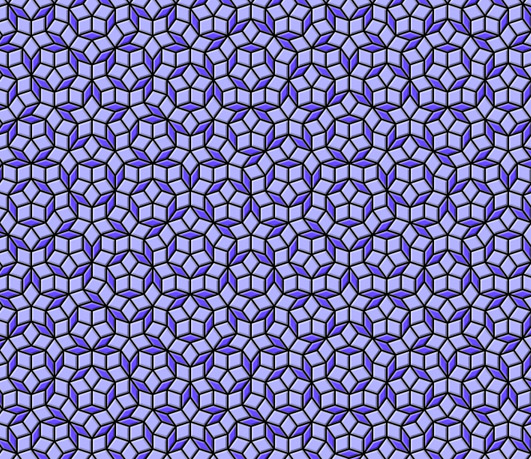# Penrose Tiling in Obfuscated Python

Who says you can’t write obfuscated Python?

Here’s a Python script which renders some Penrose tiling. Yes, this is valid Python code:

_                                 =\
"""if!
1:"e,V=100
0,(0j-1)**-.2;
v,S=.5/  V.real,
[(0,0,4      *e,4*e*
V)];w=1          -v"def!
E(T,A,              B,C):P
,Q,R=B*w+                A*v,B*w+C
*v,A*w+B*v;retur              n[(1,Q,C,A),(1,P
,Q,B),(0,Q,P,A)]*T+[(0,C            ,R,B),(1,R,C,A)]*(1-T)"f
or!i!in!_[:11]:S       =sum([E          (*x)for       !x!in!S],[])"imp
ort!cair               o!as!O;      s=O.Ima               geSurfac
e(1,e,e)               ;c=O.Con  text(s);               M,L,G=c.
move_to                ,c.line_to,c.s                et_sour
ce_rgb                a"def!z(f,a)                :f(-a.
imag,a.       real-e-e)"for!T,A,B,C!in[i       !for!i!
in!S!if!i[""";exec(reduce(lambda x,i:x.replace(chr
(i),"\n "[34-i:]),   range(   35),_+"""0]]:z(M,A
);z(L,B);z         (L,C);         c.close_pa
th()"G             (.4,.3             ,1);c.
paint(             );G(.7             ,.7,1)
;c.fil             l()"fo             r!i!in
!range             (9):"!             g=1-i/
8;d=i/          4*g;G(d,d,d,          1-g*.8
)"!def     !y(f,a):z(f,a+(1+2j)*(     1j**(i
/2.))*g)"!for!T,A,B,C!in!S:y(M,C);y(L,A);y(M
,A);y(L,B)"!c.st            roke()"s.write_t
o_png('pen                        rose.png')
"""                                       ))


When this program runs, it outputs a 1000x1000 image file to penrose.png, consisting of about 2212 Penrose tiles rendered with a 3D relief effect. Here’s a slice of the image (click to enlarge):The script requires Pycairo. It only runs on Python <= 2.7; Python 3 is not supported. It started life as a regular Python script, but in my effort to make the code more compact, I got a bit carried away.

Penrose tilings are cool because they cover the entire plane in an aperiodic way – a shifted copy of the image never matches the original. They were invented by Sir Roger Penrose after a series of attempts to tile the plane with pentagonal shapes. For an explanation of the algorithm behind this script, see my next post, Penrose Tiling Explained.

Python never got much credit as an obfuscated programming language, compared to C or Perl. It seems a contest never took place, and there aren’t too many examples of obfuscated Python on the web: You’ll find a few examples in the official Python FAQ and on various pages such as here and here. There was also a talk at PyCon 2011.

I believe this is the first example of obfuscated Python which outputs a high-resolution image. You’ll find another example in my followup post, High-Resolution Mandelbrot in Obfuscated Python. If you know of any others, let me know in the comments!